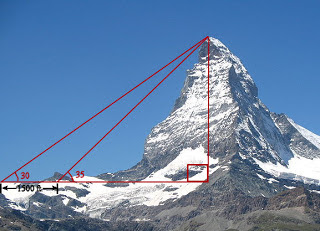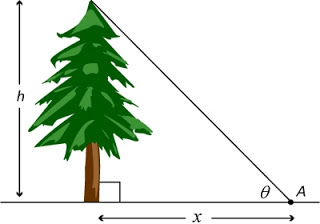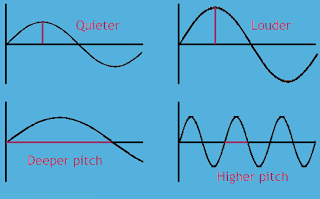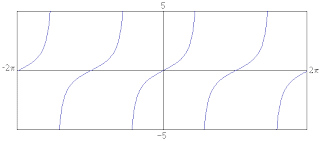## Saturday, 4 May 2013

### IntroductionThis blog tell you about trigonometry in your life. You know why we need trigonometry in our life, here some reason why it’s important:

The various fields in which trigonometry is used are acoustics, architecture, astronomy (and hence navigation, on the oceans, in aircraft, and in space; in this connection, see great circle distance), biology, cartography, chemistry, civil engineering, computer graphics, geophysics, crystallography, economics (in particular in analysis of financial markets), electrical engineering, electronics, land surveying and geodesy, many physical sciences, mechanical engineering, machining, medical imaging (CAT scans and ultrasound), meteorology, music theory, number theory (including cryptography), oceanography, optics, pharmacology, phonetics, probability theory, psychology, seismology, statistics, and visual perception, education.

From the pictures below, you can believe why trigonometry important in our life

Trigonometry is commonly used in finding the height of towers and mountains.It is used in navigation to find the distance of the shore from a point in the sea.It is used in oceanography in calculating the height of tides in oceansIt is used in finding the distance between celestial bodiesThe sine and cosine functions are fundamental to the theory of periodic functions such as those that describe sound and light waves.We also find trigonometry’s principle in the game, for example in angry birdSo, is trigonometry important? Definitely a resounding YES! Learn it, know it, use it to enhance your life. Theoretically, you could go through life completely ignorant of what trigonometry is capable of doing for you. You could have no knowledge of trigonometry, or any mathematics. Many people do. The drawback to such a life is that one is then not able to understand the wonders that nature has to offer. Mathematics is the tool by which we, as human beings, are able to understand the unfathomable beauty of the universe.

One last note on trigonometry: Although many people think trigonometry is completely divorced from algebra, it really isn't. Trigonometry is an extension of algebraic ideas—functions in particular—to trigonometric concepts. We can often treat trigonometric concepts in algebraic terms. Solving a trigonometric equation is very similar to solving an algebraic equation. Do not be confused by the changes in terminology. However, despite the similarities, there are also a number of differences. In the Trig Brain, we will attempt, wherever possible, to point out the similarities and differences between trigonometry and algebra. A thorough understanding of both is a prerequisite for higher mathematics such as calculus and differential equations.

Now, it's time to learn Trigonometry's formula....
The first thing to understand with trigonometry is why the mathematics of right-angled triangles should also be the mathematics of circles. Picture a line which can turn around one of its ends, like the hand of a clock. Obviously, the moving end of the line traces out a circle - it's like drawing with a compass. Now, consider how far this point is to the right or left of the centre point (we call this distance x), and how far above or below (which we'll call y). By attaching horizontal and vertical lines of lengths x and y to the ends of the first line we get a right-angled triangle, like this:
So the mathematical relationship between circles and the set of right-angled triangles should be clear: The position (x, y) of a point at an angle of θ around a circle of radius r is related to θ and r in exactly the same way that the lengths of the adjacent (x) and opposite (y) sides of a right-angled triangle are related to the length of the hypotenuse r and the angle θ.

Sine and Cosine This relationship is expressed by the two most fundamental equations of trigonometry: x = r × cos θ y = r × sin θ Or, equivalently: cos θ = x/r sin θ = y/r
Sin (sine) is the ratio of the vertical side (the side opposite the corner we're looking at) to the hypotenuse. Cos (cosine) is likewise the ratio of the horizontal side (the side adjacent to that corner) to the hypotenuse. Sine and cosine are functions, which is to say that they take one number (an angle in this case, usually expressed in degrees or radians) and spit out another. For certain values of θ, it is easy to figure out what the sine and cosine values are going to be just by thinking about what the angle corresponds to on the circle; the simplest cases are for θ = 0°, which is a line pointing right, giving cos θ = 1 and sine θ = 0; a line pointing straight up (ie. θ = 90°), which gives us cos θ = 0 and sine θ = 1, and so on. At 45° the opposite and adjacent sides are the same length, so from Pythagoras' Theorem (r2=x2 + y2) they must each be (√2)/2.

For values in between the sine and cosine vary in a smooth curve, so that a plot of sin x against x is your basic wavy line:Cosine is to sine as horizontal is to vertical, so the graph of cosine is just like the graph of sine shifted by one quarter-turn. On a graph together, they look like this:Tangent The third basic trigonometric function is called the tangent (tan for short), and it is defined as the ratio of the opposite and adjacent sides - that is: tan θ = y/x = sin θ/cos θ Its graph looks like this:Inverse Functions and Reciprocals More Triangles,
So far, I have only talked about trigonometry as it concerns right-angled triangles and circles. But trigonometry takes in the study of all kinds of triangles - be they equilateral, isosceles or scalene. Equilateral triangles just have three sides the same length, and three 60° corners. Isosceles triangles have two sides the same length and hence two identical angles, so it's easy to split them down the middle and treat them as two identical right-angled triangles back to back. Scalene triangles, on the other hand, have every side and angle different, so if you ever have to calculate their lengths and angles you're likely to want to use the the Sine Rule and the Cosine Rule (unless they happen to be right-angled scalene triangles, which obviously makes things easier). With three different angles to work with, it's easiest to call them A, B and C, and call the lengths of the sides opposite them a, b c. The Sine Rule can then be writtenThis is useful, for instance, if you know two angles and the length of one side of a triangle, and you need to find the length of another side; or if you know the lengths of two sides and one angle (which is not the angle between those sides), and you need to find one or more other angles. In cases where you have two sides and the angle between them, or you are given all three lengths and asked to calculate angles, you will need to switch to the Cosine Rule, which can be written in two main ways:The general formula for finding the area of a triangle is area = ½ × base × height Which is also equal to area = ½ × a × b × sin C. The choice of which angle is which in all of these equations is of course completely abritrary, so feel free to swap around a, b and c at will, as long as you also swap A, B and C to make them fit.